Function Repository Resource:

# TriangleEdgeAngles

Given edge lengths of a triangle, return the angles opposite those edges

Contributed by: Wolfram Staff
 ResourceFunction["TriangleEdgeAngles"][{a,b,c}] returns the angles opposite edges (a,b,c).

## Examples

### Basic Examples (1)

Edges of a 30-60-90 triangle:

 In:=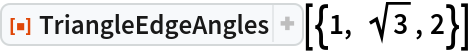Out=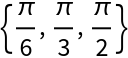### Scope (2)

Edges for a 45-60-75 look complicated:

 In:=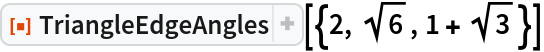Out=RootReduce can help:

 In:=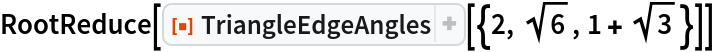Out=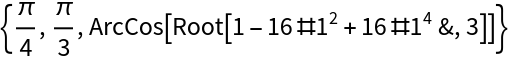In:=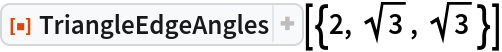Out=## Requirements

Wolfram Language 11.3 (March 2018) or above

## Version History

• 1.0.0 – 11 February 2019